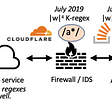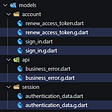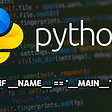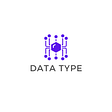# Best Time to Buy and Sell Stock with Transaction Fee — Day 97(Python)

Today’s question is from the Daily Leetcode coding challenge — March edition. Let us look into the problem statement.

714. Best Time to Buy and Sell Stock with Transaction Fee

You are given an array `prices` where `prices[i]` is the price of a given stock on the `ith` day, and an integer `fee` representing a transaction fee.

Find the maximum profit you can achieve. You may complete as many transactions as you like, but you need to pay the transaction fee for each transaction.

Note: You may not engage in multiple transactions simultaneously (i.e., you must sell the stock before you buy again).

Example 1:

`Input: prices = [1,3,2,8,4,9], fee = 2Output: 8Explanation: The maximum profit can be achieved by:- Buying at prices = 1- Selling at prices = 8- Buying at prices = 4- Selling at prices = 9The total profit is ((8 - 1) - 2) + ((9 - 4) - 2) = 8.`

Example 2:

`Input: prices = [1,3,7,5,10,3], fee = 3Output: 6`

Constraints:

• `1 < prices.length <= 5 * 104`
• `0 < prices[i], fee < 5 * 104`

We have an array of stock prices and a fee which is the amount to be paid when we perform a transaction. A transaction would mean we buy and then sell the stock, in such a case a fee will be charged.

For each price, we can buy if we do not hold any stock or sell if we hold any stock or do absolutely nothing.

If we have to solve this problem using recursion, we require a variable that can flag if we are holding a stock earlier. This flag variable will ensure we do not sell any stock if we are not holding one or We avoid buying stock if we are already holding some stock.

If we have already bought stocks, we would have two options; either do nothing or sell stocks at the current price and include the fee.

If we do not have any stock in hand currently, we have two options; either do nothing or buy stocks at the current price.

Let us look at the recursive code snippet.

`class MaxProfitFinder:    def maxProfit(self, prices: List[int], fee: int) -> int:        def cost(i, n, bought_prev):            if i >= n:                return 0                if bought_prev == True:                return max(cost(i+1, n, False) + prices[i] - fee, cost(i+1, n, bought_prev))            else:                return max(cost(i+1, n, True) - prices[i], cost(i+1, n, bought_prev))                    return cost(0, len(prices), False)`

To memoize the above code, we can make use of a dictionary. The key of the dictionary will be the index of the current price in the array and flag that mentions if we bought or sold the current stock. The value will be the profit that we will earn.

`import collectionsclass MaxProfitFinder:    def maxProfit(self, prices: List[int], fee: int) -> int:        dp = defaultdict()        def cost(i, n, bought_prev):            if i >= n:                return 0            if (i,bought_prev) in dp:                return dp[(i,bought_prev)]                        if bought_prev == True:                dp[(i,bought_prev)] = max(cost(i+1, n, False) + prices[i] - fee, cost(i+1, n, bought_prev))            else:                dp[(i,bought_prev)] = max(cost(i+1, n, True) - prices[i], cost(i+1, n, bought_prev))            return dp[(i,bought_prev)]        return cost(0, len(prices), False)`

# Complexity analysis.

Time Complexity

The time complexity is O(2N) since we are traversing through our price array twice; once if we buy the stock and second if we are not buying that stock. Since O(2N) can be represented as O(N). The actual time complexity is O(N).

Space Complexity

The space complexity is O(2N) since we are saving the results in the form of a dictionary. Since O(2N) can be represented as O(N). The actual space complexity is O(N).

--

--

--

## More from Annamariya Tharayil

Software Engineer. Find me @ www.linkedin.com/in/annamariya-jt

Love podcasts or audiobooks? Learn on the go with our new app.

## The Regular Expression Denial of Service (ReDoS) cheat-sheet## Flutter Minimalist: App Account Management Part-2(not Firebase)## What’s the Scrum method ?## Extension methods in C### Tech for Non-tech in Okayama, Japan## Makers vs Managers## Annamariya Tharayil

Software Engineer. Find me @ www.linkedin.com/in/annamariya-jt

## Digging through Jeopardy! Questions using Python and Natural Language Processing## Python if __name__ = ‘__main__’ explained## What am I using type data in python?